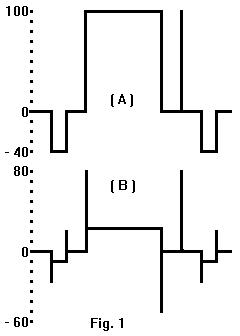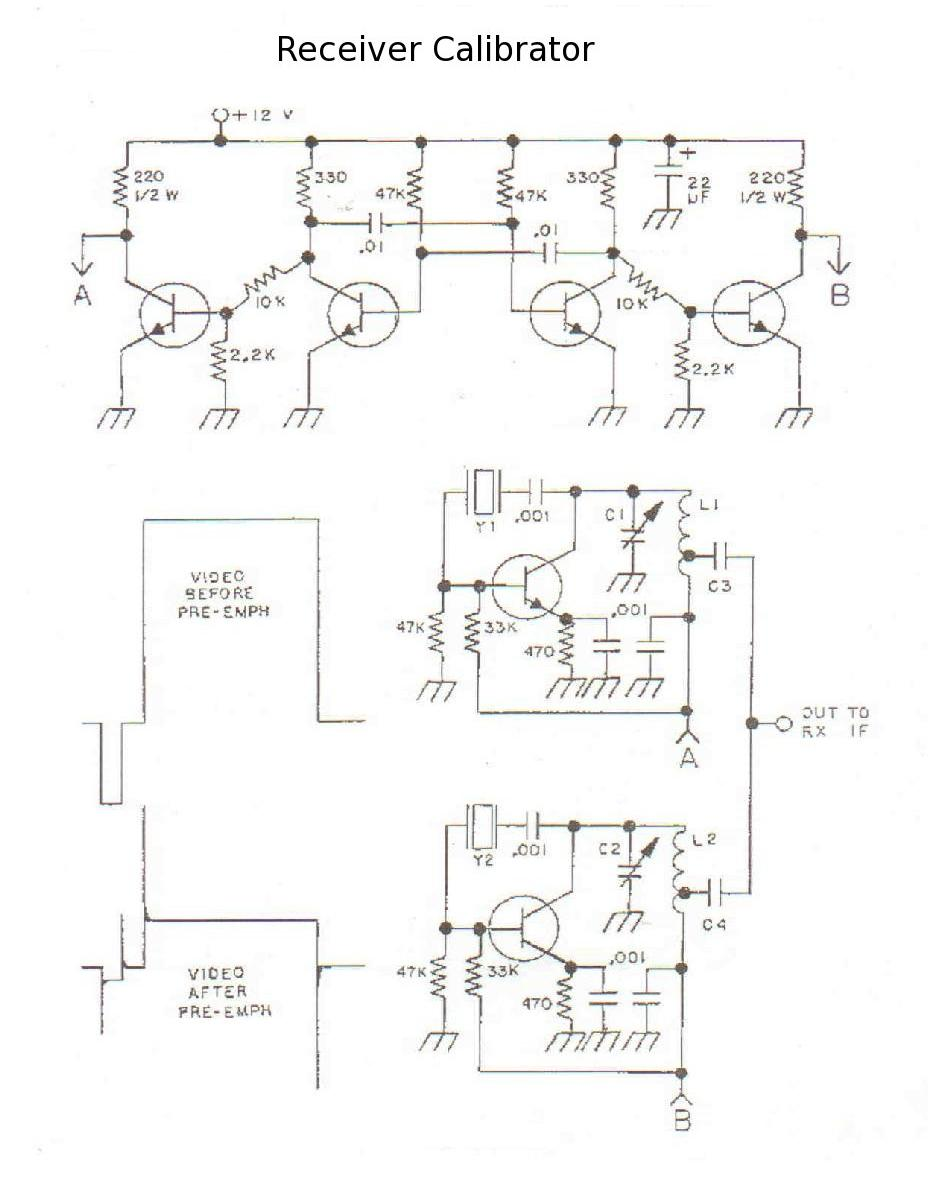### of an FM TV Receiver IF system to measure video deviation

The following is the draft of my original article which was published in Amateur Television Quarterly (ATVQ) - Spring 1994.The measurement of pre-emphasized video deviation is based on the total peak-to-peak amplitude of the pre-emphasized video compared to the ratio of the amplitude at white. See the drawing for comparative levels of pre-emphasized video and assume commercial standards of 125 nanoseconds for rise and fall times to and from white and CCIR 405 pre-emphasis.

The low frequency white bar is attenuated by 12.92 db while the high frequency rise and fall times are increased in amplitude by 2.92 db by the pre-emphasis network. The total difference is 15.85 db (a factor of 6.20) between total peak-to-peak amplitude and the amplitude of white to blanking. We can measure the composite video and infer the total pre-emphasized levels - assuming correct pre-emphasis at the proceeding FM TV transmitter.

The calibrator substitutes a square wave of known deviation for the video allowing calculation of a calibration factor for the receiver de-emphasized video output. The accuracy of the receiver de-emphasis and video frequency response is not too important in this case since calibration and measurement are both done at the low frequency end of the base band range. Measurement errors will mostly be caused by frequency response inaccuracies at the proceeding FM TV transmitter.

The calibrator circuit (shown at the end of this article) consists of two crystal controlled overtone oscillators alternately switched ON by a multi vibrator timing and switching circuit. Crystals (surplus OK) should be chosen approximately 1 to 1.5 Mhz apart in frequency and their average frequency should approximately correspond to your receiver IF center frequency. The average frequency is not too critical assuming the receiver IF has a flat RF band pass through the two switched frequencies.

The calibrator which I use has crystals of 71.245 and 69.745 Mhz for an average frequency of 70.495 Mhz for use with a 70 Mhz IF system. The oscillator tank inductors (L1 & L2) are each eight turns of number 22 enameled wire spaced one wire diameter and formed on a 1/4 inch drill bit. Tuning capacitors (C1 & C2) are 6-50 pf trimmers which resonate at approx midrange with the above crystals. The transistors in my unit are all 2N3904 and anything similar should work. The oscillators use a very high level of feedback to insure fast starting at the switching rate and a good quality square wave at the receiver video output. Oscillator output capacitors (C3 & C4) are identical wire gimmicks of 1 or 2 pf tapped down on the tank coils at 2 turns from the ground end.

Calibrator adjustment is performed by feeding it's output into the receiver IF system and using wire cutters trim the C3 & C4 wire gimmicks the same amount for correct RF level into your IF system. Tune C1 & C2 for best square waveform as observed on an oscilloscope connected to the receiver video output. The calibrator switches frequencies at a rate of approximately 2860 hz which should be well above the low frequency limitations of some video amplifiers.

Receiver calibration is done by measuring the peak-to-peak amplitude of the square wave (ignore any overshoot) at the receiver de-emphasized video output and calculating the calibration factor using the formula:

Calibration Factor = (Fh - Fl) (6.2 / 2) (0.714 / sq w)

Where:

Calibration Factor = peak deviation in Mhz per volt of composite video.
(Fh - Fl) = frequency difference between the two calibrator crystals.
sq w = peak-to-peak amplitude of the square wave in volts.

Operational measurements of pre-emphasized video deviation are made by measuring the total peak-to-peak amplitude of the composite video (sync tip to white) in volts and multiplying by the receiver calibration factor. Some cautions are in order at this point. The video must contain white. You may have to watch it for a while on the oscilloscope to determine the actual white level. Video-to sync ratio must also be correct. If not, measure the level from blanking to white and multiply by 1.4 to get a corrected composite video amplitude to multiply by the calibration factor.

Subcarrier deviation may also be measured if your calibrated de-emphasized test point carries them. Use the same calibration factor as for composite video. The frequency response of your de-emphasis network and video amplifiers now become important because measurement is done at the high frequency end of base band range but calibration was done at the low end. Filter out one subcarrier at a time for peak-to-peak amplitude measurement on the oscilloscope and correct this level by the filter attenuation factor before multiplying by the receiver deviation calibration factor. Subcarrier deviations will actually be a few percent higher than indicated due to their placement of the pre-emphasis curve.

The calibration of a FM TV receiver to measure deviation of video which has not been pre-emphasized and the calibration of a video test point ahead of the de-emphasis network are both the same procedure. The calibrator (Fh - Fl) gives a reference peak-to-peak deviation level and the receiver peak-to-peak calibration factor is obtained by direct proportion. Divide the peak-to-peak factor by 2 to get the peak deviation receiver calibration factor. This simple calibrator is a lot easier to use than setting up all the necessary test equipment to do a Bessel carrier null calibration on your FM TV receiver. I hope everyone finds it as useful as I have.

Please send E-Mail to: mathison (aatt) sdf-eu.org
.
Last revision 2012-12-15